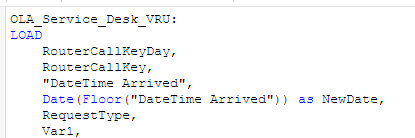# New to Qlik Sense

Discussion board where members can get started with Qlik Sense.

Announcements
Qlik® Product Spotlight: Discover what’s possible. Get more from our products.
See for yourself. Register today.
Contributor II

## Date range is not working properly

Hi All,

My source is excel with only one date field. I need to implement From Date and To Date in dashboard.

As per data :

Count(CallTypeID) = 369 in 14th Oct

Count(CallTypeID) = 1 in 15th Oct

Now in Qlik Dashboard if I select :

From Date= 14th and To Date = 14th, then count = 369

From Date= 14th and To Date = 15th, then count = 370 as expected. But if I select

From Date= 15th and To Date = 15th, then count = 370, however it should be 1.

My expression is below:

Count({\$<NewDate={'>=\$(=\$(v_FromDate))'},NewDate={'<=\$(=\$(v_ToDate))'}>}[CallTypeID]).

Thanks,

Sarif

1 Solution

Accepted SolutionsMVP & Luminary

## Re: Date range is not working properly

Hi,

You have to create variables in the front end

Document Properties -> Variable Overview and add variables

v_FromDate = Date(Min(From_Date))

v_ToDate  = Date(Max(To_Date))

Now in chart use below expression.

Count({\$<NewDate={'>=\$(=v_FromDate)<=\$(v_ToDate)'}>}[CallTypeID])

Hope this help syou.

Regards,

Jagan.

6 Replies
Valued Contributor II

## Re: Date range is not working properly

Try like this:

Count({\$<NewDate={'>=\$(=(v_FromDate))'},NewDate={'<=\$(=(v_ToDate))'}>}[CallTypeID])MVP & Luminary

## Re: Date range is not working properly

Hi,

Try like this

Count({\$<NewDate={'>=\$(=v_FromDate)'},NewDate={'<=\$(v_ToDate)'}>}[CallTypeID])

Note: The date format of variables and the NewDate dimension should be same format.  Also the NewDate field should be a date field.

Regards,

Jagan.

Contributor II

## Re: Date range is not working properly

Not working sir,

Yes NewDate and variable is on same date.Date_From:

Date(Floor("DateTime Arrived")) as From_Date

Resident OLA_Service_Desk_VRU;

Date_To:

Date(Floor("DateTime Arrived")) as To_Date

Resident OLA_Service_Desk_VRU;

Set v_FromDate = From_Date;

Set v_ToDate = To_Date;

Thanks,

SarifMVP & Luminary

## Re: Date range is not working properly

Hi,

You have to create variables in the front end

Document Properties -> Variable Overview and add variables

v_FromDate = Date(Min(From_Date))

v_ToDate  = Date(Max(To_Date))

Now in chart use below expression.

Count({\$<NewDate={'>=\$(=v_FromDate)<=\$(v_ToDate)'}>}[CallTypeID])

Hope this help syou.

Regards,

Jagan.

Contributor II

## Re: Date range is not working properly

What is the difference between those variables? If I create in Script and Front End?MVP & Luminary

## Re: Date range is not working properly

Hi,

Your statements wont work.

Set v_FromDate = From_Date;

Set v_ToDate = To_Date;

You have to change like this, it is not possible to assign a field value, it just stores as a string.

LET vFromDate = Peek('From_Date');

LET v_ToDate = Peek('To_Date');

Is that front end one working?

Regards,

Jagan.

Community Browser